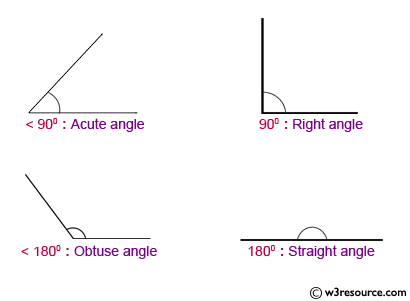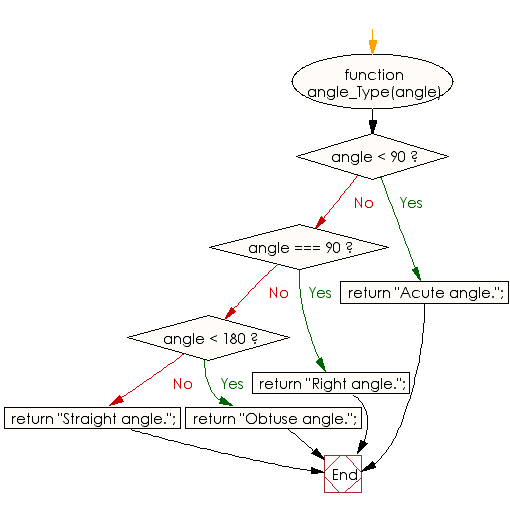﻿ JavaScript basic: Find the types of a specified angle - w3resource# JavaScript: Find the types of a specified angle

## JavaScript Basic: Exercise-86 with Solution

Write a JavaScript program to find the types of a given angle.

Types of angles:
• Acute angle: An angle between 0 and 90 degrees.
• Right angle: An 90 degree angle.
• Obtuse angle: An angle between 90 and 180 degrees.
• Straight angle: A 180 degree angle.

Pictorial Presentation:Sample Solution:

HTML Code:

``````<!DOCTYPE html>
<html>
<meta charset="utf-8">
<meta name="viewport" content="width=device-width">
<title>Find the types of a specified array</title>
<body>

</body>
</html>
```
```

JavaScript Code:

``````function angle_Type(angle) {
if(angle < 90) {
return "Acute angle.";
}
if(angle === 90) {
return "Right angle.";
}
if(angle < 180) {
return "Obtuse angle.";
}
return "Straight angle.";
}

console.log(angle_Type(47))
console.log(angle_Type(90))
console.log(angle_Type(145))
console.log(angle_Type(180))
```
```

Sample Output:

```Acute angle.
Right angle.
Obtuse angle.
Straight angle.
```

Flowchart:ES6 Version:

``````function angle_Type(angle) {
if(angle < 90) {
return "Acute angle.";
}
if(angle === 90) {
return "Right angle.";
}
if(angle < 180) {
return "Obtuse angle.";
}
return "Straight angle.";
}

console.log(angle_Type(47))
console.log(angle_Type(90))
console.log(angle_Type(145))
console.log(angle_Type(180))
``````

Live Demo:

See the Pen javascript-basic-exercise-85 by w3resource (@w3resource) on CodePen.

What is the difficulty level of this exercise?

Test your Programming skills with w3resource's quiz.

﻿

## JavaScript: Tips of the Day

Checks if a string is an anagram of another string (case-insensitive, ignores spaces, punctuation and special characters)

Example:

```const isAnagram = (str1, str2) => {
const normalize = str =>
str
.toLowerCase()
.replace(/[^a-z0-9]/gi, '')
.split('')
.sort()
.join('');
return normalize(str1) === normalize(str2);
};
console.log(isAnagram('iceman', 'cinema')); // true
```

Output:

```true
```